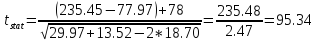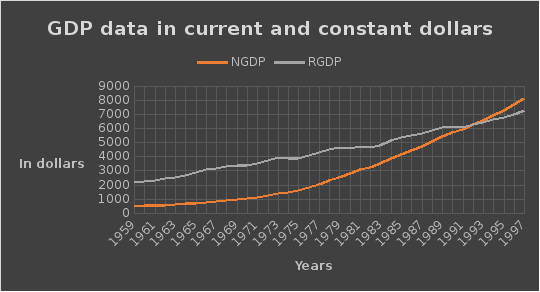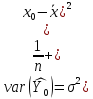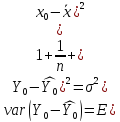# From the linear model we find expected u

 Using this formula we find out the tstatOur tcrit is 2.052 (df is 27, α is 0.05) tstat value is greater than tcrit, which means that we reject the H0 and conclude that the sum of the coefficients of X1,i and X2,i is not negative 78. Partial coefficient of correlation is obtained due to the reason that we need a coefficient of correlation that is free of influence, if any, of X3 on X2 and Y. Also, it can be defined as following: r1 2.3 = PCC between Y and X2, holding X3 constant r1 3.2 = PCC between Y and X3, holding X2 constant r2 3.1 = PCC between X2 and X3, holding Y constant Question 4NGDPt= -986.3317 +201.9772Tt + ut SE= 212.6816 9.267491 R2 = 0.9277 t= -4.64 21.79 RGDPt= 1907.715 +128.782Tt + ut SE= 45.13298 1.966646 R2 = 0.9914 t= 42.27 65.48 On average, the nominal GDP of USA has increased 202 USD annually starting from 1959 year. On average, the real GDP of USA has increased 129 USD annually starting from 1959 year. There is a difference between the coefficients of current and constant dollar GDP. The difference is on average is about 73 USD. This difference can be explained by the inflation that is included in RGDP. The graph and regression analysis shows that, nominal GDP is increasing in a faster pace compared to the real one. This can be explained by the fact that RGDP is adjusted to the inflation and the rate of inflation in USA is increasing. Question 5 According to the regression analysis, the linear model has been constructed: Payi = 12129.52 + 3.307597Spendi + ui SE= 1197.408 0.3117191 R2=0.6968 RSS=264850534 SSE=608559605 Ho: β₂=3.0 Ha: β₂≠3.0 β₂=3.307597± 2*(0.3117191) => β₂= [2.68411588; 3.9310352] => this is the 95% confidence interval the interval shows that the coefficient of a slope is inside this interval with a 95% confidence. Individual and mean forecast values when spending is 5000\$ is Payi = 12129.52 + 3.307597*5000 = =28679.63452 Standard error of the mean value is 520.5117, which is found by the formulaStandard error of the individual value is 2382.337, which is found by the formulaThen the confidence intervals for mean individual predictions are calculated:Download 0.84 Mb.Do'stlaringiz bilan baham: